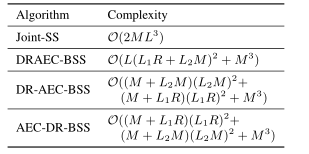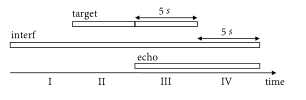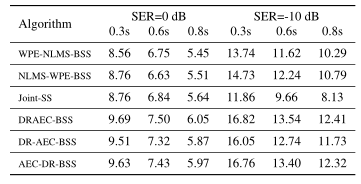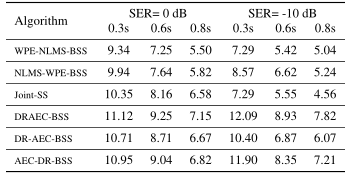# 联合在线多通道声学回声消除、语音去混响和声源分离

### 摘要：

本文提出了一种联合声源分离算法，可同时减少声学回声、混响和干扰源。通过最大化相对于其他源的独立性，将目标语音从混合中分离出来。结果表明，分离过程可以分解为级联的子过程，分别与声学回声消除、语音去混响和源分离相关，所有这些都使用基于辅助函数的独立分量/矢量分析技术及其求解顺序来求解是可交换的。级联解决方案不仅导致较低的计算复杂度，而且比普通联合算法具有更好的分离性能。

### 1引言

在全双工语音交互模式下工作的智能设备需要同时处理回放回声、房间混响和干扰源。文献中对这三种失真进行了广泛的研究，并且已经分别开发了许多经典算法，例如用于声学回声消除 (AEC) 的归一化最小均方 (NLMS) 算法 [1, 2]、加权预测误差 (WPE) ) 算法 [3, 4] 用于语音去混响 (DR) 和基于辅助功能的独立分量/矢量分析 (Aux-ICA/IVA) 算法 [5, 6] 用于盲源分离 (BSS)。考虑两种或三种失真的联合解决方案很有吸引力，特别是对于现实世界的应用，并且可以带来优于单独算法的性能改进 [7, 8, 9, 10, 11, 12, 13, 14, 15, 16, 17, 18]。

Takeda等人[7, 8] 通过应用频域 ICA 的分离模型来实现盲去混响和回声消除，该模型使用在多个输入/输出逆滤波定理 (MINT) 条件下保持的观察到的信号独立性。作者还开发了一些技术来降低他们的可驳接机器人应用程序的计算成本。

Yoshioka等人  提出了一种条件分离和去混响 (CSD) 方法，其中分离和预测矩阵交替优化，相互依赖。Boeddeker等人  提出了一种加权功率最小化无失真响应 (WPD) 波束形成器，可以同时执行去噪和去混响。该波束形成器在单一似然最大化标准下进行了优化，并显示出优于传统 WPE 级联和最小功率无失真响应 (MPDR) 波束形成器的优势。

已经提出了几种联合方法来同时执行 AEC、DR 和 BSS [16, 17]。 Togami 和 Kawaguchi  通过假设麦克风输入信号的时变局部高斯模型，将语音去混响、降噪和声学回声降低结合在一个统一的框架中。基于期望最大化方法迭代优化算法参数以计算期望信号的最小均方误差估计。Carbajal等人  进一步引入了一个神经网络来模拟回声消除和去混响后目标和残余信号的短期频谱。

我们之前的工作 [19, 20] 分别重新审视了 DR 和 AEC 的问题，并提出了基于 Aux-ICA 的源分离方法。本文进一步提出从统一的源分离角度联合执行 AEC、DR 和 BSS，假设混合源相互独立。首先提出了一种联合源分离算法，但其计算成本很高。然后我们启发式地分解分离矩阵，并将联合优化问题划分为可以顺序处理的子问题。由于放宽了联合算法中所做的假设，顺序级联解决方案不仅降低了计算复杂度，而且具有更好的分离性能。

本文的其余部分安排如下。在第 2 节中，我们使用卷积信号模型来表述问题。第 3 节介绍了联合源分离算法和级联解决方案。第 4 节和第 5 节分别给出了实验和结论。

### 2问题表述

我们考虑短时傅里叶变换 (STFT) 域中的多通道卷积混合。 M 个传感器阵列捕获来自 N 个近端源$$\mathbf{s}=\left[s_{1}, s_{2}, \ldots, s_{N}\right]^{T}$$$$R$$个远端源$$\mathbf{r}=\left[r_{1}, r_{2}, \ldots, r_{R}\right]^{T}$$的信号，其中$$(\cdot)^{T}$$表示转置。传感器信号$$\mathbf{x}=\left[x_{1}, x_{2}, \ldots, x_{M}\right]^{T}$$由下式给出：

$\mathbf{x}(t, f)=\sum_{l=0}^{\infty} \mathbf{A}_{l} \mathbf{s}(t-l, f)+\sum_{l=0}^{\infty} \mathbf{B}_{l} \mathbf{r}(t-l, f) (1)$

为了提取近端源的直接路径和早期反射，信号模型 (1) 可以近似转换为自回归模型 [8, 16]，如下所示：

$\mathbf{x}(t)=\mathbf{A}_{0} \mathbf{s}(t)+\overline{\mathbf{B}} \overline{\mathbf{r}}(t)+\overline{\mathbf{C}} \overline{\mathbf{x}}(t-\Delta) (2)$

\begin{aligned} \overline{\mathbf{B}} &=\left[\mathbf{B}_{0}, \mathbf{B}_{1}, \ldots, \mathbf{B}_{L_{1}-1}\right], \\ \overline{\mathbf{r}}(t) &=\left[\mathbf{r}(t), \mathbf{r}(t-1), \ldots, \mathbf{r}\left(t-L_{1}+1\right)\right]^{T} \\ \overline{\mathbf{C}} &=\left[\mathbf{C}_{0}, \mathbf{C}_{1}, \ldots, \mathbf{C}_{L_{2}-1}\right] \\ \overline{\mathbf{x}}(t-\Delta) &=\left[\mathbf{x}(t-\Delta), \ldots, \mathbf{x}\left(t-\Delta-L_{2}+1\right)\right]^{T}, \end{aligned} (3)

L1、L2 是传递函数的阶数。 (2) 的矩阵符号由下式给出：

$\left[\begin{array}{c} \mathbf{x}(t) \\ \overline{\mathbf{r}}(t) \\ \overline{\mathbf{x}}(t-\Delta) \end{array}\right]=\left[\begin{array}{ccc} \mathbf{A}_{0} & \overline{\mathbf{B}} & \overline{\mathbf{C}} \\ \mathbf{0} & \mathbf{I}_{1} & 0 \\ 0 & 0 & \mathbf{I}_{2} \end{array}\right]\left[\begin{array}{c} \mathbf{s}(t) \\ \overline{\mathbf{r}}(t) \\ \overline{\mathbf{x}}(t-\Delta) \end{array}\right] (4)$

$\left[\begin{array}{c} \mathbf{s}(t) \\ \overline{\mathbf{r}}(t) \\ \overline{\mathbf{x}}(t-\Delta) \end{array}\right]=\underbrace{\left[\begin{array}{ccc} \mathbf{D} & \mathbf{E} & \mathbf{F} \\ 0 & \mathbf{I}_{1} & 0 \\ \mathbf{0} & \mathbf{0} & \mathbf{I}_{2} \end{array}\right]}_{\mathbf{w}}\left[\begin{array}{c} \mathbf{x}(t) \\ \mathbf{r}(t) \\ \overline{\mathbf{x}}(t-\Delta) \end{array}\right] (5)$

进一步假设源信号的时间无关性，如 [4, 7, 8] 中所讨论的，我们可以使用$$\{\mathbf{s}(t), \overline{\mathbf{r}}(t), \overline{\mathbf{x}}(t-\Delta)\}$$是相互独立的条件。因此，可以通过最小化 Kullback-Leibler 散度 (KLD) 来半盲估计分离矩阵$$\mathbf{W}$$

$J(\mathbf{W})=\int p(\mathbf{s}, \overline{\mathbf{r}}, \overline{\mathbf{x}}) \log \frac{p(\mathbf{s}, \overline{\mathbf{r}}, \overline{\mathbf{x}})}{q(\mathbf{s}) q(\overline{\mathbf{r}}) q(\overline{\mathbf{x}}))} d \mathbf{s} d \overline{\mathbf{r}} d \overline{\mathbf{x}} (6)$

### 3提出的算法

#### 3.1联合源分离

最小化 (6) 是一个非凸优化问题，可以应用基于辅助函数的技术，而不是最标准的自然梯度方法 [5, 6]。以下联合源分离算法是先前仅针对 BSS 的工作的直接扩展，但之前未进行过研究。联合算法要求混合源遵循超高斯或广义高斯 PDF，这是对语音源的有效假设，源 PDF 表示为：

$p(s) \propto \exp \left[-\left(\frac{|s|}{\lambda}\right)^{\gamma}\right] (7)$

基于(7)，辅助函数$$J(\mathbf{W}, \mathbf{V})$$设计为

$J(\mathbf{W})=\min _{\mathbf{V}} \mathbf{J}(\mathbf{W}, \mathbf{V}) (8)$

$J(\mathbf{W})=\min _{\mathbf{V}} \mathbf{J}(\mathbf{W}, \mathbf{V}) (9)$

$$(\cdot)^{H}$$表示厄米特转置。$$\mathbf{w}_{m}^{H}$$$$\mathbf{W}$$的第$$m$$行向量，引入的辅助变量

$\mathbf{V}_{m}=\mathbb{E}\left[\beta_{m}(t) \mathbf{u}(t) \mathbf{u}^{H}(t)\right] (10)$

$$\mathbb{E}$$是期望算子，$$\mathbf{u}(t)=[\mathbf{s}(t), \overline{\mathbf{r}}(t), \overline{\mathbf{x}}(t-\Delta)]^{T}$$，源 PDF 相关权重因子

$\beta_{m}(t)=\left(\sum_{f}\left|\hat{s}_{m}(t)\right|^{2}\right)^{\frac{\gamma-2}{2}} (11)$

$\overrightarrow{8}_{m}(t)=w_{m}^{H} \mathbf{1 1}(t) (12)$

\begin{aligned} &\mathbf{w}_{m}=\left(\mathbf{W} \mathbf{V}_{m}\right)^{-1} \mathbf{i}_{m,}, \\ &\mathbf{w}_{m}=\frac{\mathbf{w}_{m}}{\sqrt{\mathbf{w}_{m}^{H} \mathbf{V}_{m} \mathbf{w}_{m}}} \end{aligned} (13)

#### 3.2级联解决方案

联合算法的计算复杂性一直受到关注 [7, 12, 14]。 (13) 中的计算涉及矩阵乘法和 $$\mathcal{O}\left(L^{3}\right)$$ 阶矩阵求逆，其中 $$L=M+L_{1} R+L_{2} M$$，这对于实际应用来说在计算上可能相当昂贵。一种直观的方法是将大的分离矩阵$$\mathbf{W}$$分解为可以更有效地求解的较小部分。

$$\mathbf{W}$$的等价形式由下式给出：

$\mathbf{W}_{1}=\underbrace{\left[\begin{array}{ccc} \mathrm{D} & 0 & \mathbf{0} \\ \mathbf{0} & \mathbf{I}_{1} & 0 \\ \mathbf{0} & 0 & \mathbf{I}_{2} \end{array}\right]}_{\mathbf{W}_{\mathrm{BSS}}} \underbrace{\left[\begin{array}{ccc} \mathbf{I}_{3} & \overline{\mathbf{E}} & \overline{\mathbf{F}} \\ 0 & \mathbf{I}_{1} & 0 \\ 0 & 0 & \mathbf{I}_{2} \end{array}\right]}_{\mathbf{W}_{\mathrm{DRAEC}}} (14)$

$\mathbf{W}_{2}=\underbrace{\left[\begin{array}{ccc} \mathbf{D} & \mathbf{0} & 0 \\ \mathbf{0} & \mathbf{I}_{1} & 0 \\ \mathbf{0} & \mathbf{0} & \mathbf{I}_{2} \end{array}\right]}_{\mathbf{W}_{\mathrm{BSS}}} \underbrace{\left[\begin{array}{ccc} \mathbf{I}_{3} & \overline{\mathbf{E}} & 0 \\ 0 & \mathbf{I}_{1} & 0 \\ 0 & 0 & \mathbf{I}_{2} \end{array}\right]}_{\mathbf{W}_{\text {AEC }}} \underbrace{\left[\begin{array}{ccc} \mathbf{I}_{3} & \mathbf{0} & \overline{\mathbf{F}} \\ \mathbf{0} & \mathbf{I}_{1} & 0 \\ 0 & 0 & \mathbf{I}_{2} \end{array}\right]}_{\mathbf{W}_{\mathrm{DR}}} (15)$

$\mathbf{W}_{3}=\underbrace{\left[\begin{array}{ccc} \mathbf{D} & 0 & 0 \\ 0 & \mathbf{I}_{1} & 0 \\ 0 & 0 & \mathbf{I}_{2} \end{array}\right]}_{\mathbf{W}_{\mathrm{BSS}}} \underbrace{\left[\begin{array}{ccc} \mathbf{I}_{3} & 0 & \overline{\mathbf{F}} \\ 0 & \mathbf{I}_{1} & 0 \\ 0 & 0 & \mathbf{I}_{2} \end{array}\right]}_{\mathbf{W}_{\mathrm{DR}}} \underbrace{\left[\begin{array}{ccc} \mathbf{I}_{3} & \overline{\mathbf{E}} & 0 \\ 0 & \mathbf{I}_{1} & 0 \\ 0 & 0 & \mathbf{I}_{2} \end{array}\right]}_{\mathbf{W}_{\mathrm{AEC}}} (16)$

上述矩阵分解将(5)中的分离过程转化为与AEC、DR和BSS自然相关的子过程，可以顺序求解，而不是联合求解。请注意，BSS 的求解顺序不是放在第一位的，因为它会导致未确定的源分离子问题。

#### 3.3顺序更新技术

着眼于AEC-DR-BSS算法中的分离矩阵(16)，我们首先将$$\mathbf{W}_{\text {AEC }}$$带入(5)，有

$\left[\begin{array}{l} \mathbf{y}(t) \\ \overline{\mathbf{r}}(t) \end{array}\right]=\left[\begin{array}{cc} \mathbf{I}_{3} & \overline{\mathbf{E}} \\ \mathbf{0} & \mathbf{I}_{1} \end{array}\right]\left[\begin{array}{l} \mathbf{x}(t) \\ \overline{\mathbf{r}}(t) \end{array}\right] (17)$

$\overline{\mathbf{E}}=-\mathbf{Q}_{\text {AEC }} \mathbf{V}_{\mathrm{AEC}}^{-1} (18)$

\begin{aligned} &\mathbf{Q}_{\mathrm{AEC}}=\mathbb{E}\left[\beta_{\mathrm{AEC}}(t) \mathbf{x}(t) \overline{\mathbf{r}}^{H}(t)\right] \\ &\mathbf{V}_{\mathrm{AEC}}=\mathbb{E}\left[\beta_{\mathrm{AEC}}(t) \overline{\mathbf{r}}(t) \overline{\mathbf{r}}^{H}(t)\right] \end{aligned} (19)

$\beta_{\mathrm{AEC}}(t)=|\hat{\mathbf{y}}(t)|^{\gamma-2} (20)$

$\hat{\mathbf{y}}(t)=\mathbf{x}(t)+\overline{\mathbf{E}} \overline{\mathbf{r}}(t) (21)$

$\left[\begin{array}{c} \mathbf{z}(t) \\ \overline{\mathbf{y}}(t-\Delta) \end{array}\right]=\left[\begin{array}{cc} \mathbf{I}_{3} & \overline{\mathbf{F}} \\ 0 & \mathbf{I}_{2} \end{array}\right]\left[\begin{array}{c} \mathbf{y}(t) \\ \overline{\mathbf{y}}(t-\Delta) \end{array}\right] (22)$

$\overline{\mathbf{F}}=-\mathbf{Q}_{\mathrm{DR}} \mathbf{V}_{\mathrm{DR}}^{-1} (23)$

\begin{aligned} &\mathbf{Q}_{\mathrm{DR}}=\mathbb{E}\left[\beta_{\mathrm{DR}}(t) \mathbf{y}(t) \overline{\mathbf{y}}^{H}(t-\Delta)\right] \\ &\mathbf{V}_{\mathrm{DR}}=\mathbb{E}\left[\beta_{\mathrm{DR}}(t) \mathbf{y}(t-\Delta) \mathbf{y}^{H}(t-\Delta)\right] \end{aligned} (24)

$\beta_{\mathrm{DR}}(t)=|\hat{z}(t)|^{\gamma-2} (25)$

$\hat{\mathbf{z}}(t)=\hat{\mathbf{y}}(t)+\overline{\mathbf{F}} \overline{\mathbf{y}}(t-\Delta) (26)$

最后，通过将 Aux-IVA 应用于以下问题，获得$$\mathrm{D}$$的分离系数

$\mathbf{s}(t)=\mathbf{D}_{\mathbf{z}}(t) (27)$

现在可以同样推导出 DRAEC-BSS 和 DR-AEC-BSS 算法。请注意，当使用 (17) 求解 $$\overline{\mathbf{E}}$$、使用 (22) 求解$$\overline{\mathbf{F}}$$和使用 (27) 求解$$\mathrm{D}$$时，先前的声学回声、后期混响和干净声源的相互独立假设被放宽为成对独立。

鉴于上述描述，我们的算法在线实现涉及自相关矩阵$$\mathrm{V}$$、互相关矩阵$$\mathrm{Q}$$和加权因子$$\beta$$的递归估计，使用 0.999 的平滑系数$$\mathrm{C}$$。为了清楚起见，源代码可在https://github.com/nay0648/unified2021获得。

#### 3.4复杂性分析

与朴素的联合源分离 (Joint-SS) 算法相比，级联解决方案明显降低了总体计算成本。所提出算法的复杂度顺序比较如表1所示。### 4实验

#### 4.1设置

我们考虑一个用户在客厅环境中与智能扬声器交互的场景。房间大小是随机采样的，长度为 [4.0, 8.0] 米，宽度为 [3.0, 6.0] 米，高度为 [2.5, 4.0] 米。将 M = 2 个麦克风间距为 10 厘米的麦克风阵列放置在房间内，同时与墙壁保持 50 厘米的最小距离。播放回声的 R = 1 扬声器放置在传感器阵列下方 15 厘米处。用户和一个干扰源 (N = 2) 随机位于房间内。使用图像方法  生成相应的房间脉冲响应。

测试话语是按照  中的设置准备的。具体来说，每个话语都有四个 5 秒的片段，用户的语音、干扰和回声重叠，如图 1 所示。输入信号干扰比 (SIR) 设置为 0 dB，信号回声比设置为(SER) 设置为 {0,-10} dB。分离的用户语音的整体质量通过第三段中的信号失真比 (SDR) [24, 25] 来衡量。引入了两个非指导性指标，即信号加干扰加回波与干扰加回波比（SIER）和信号加干扰加干扰比（SIIR）来衡量非目标降低性能。 SIER 粗略估计为段 III 中的信号能量与段 IV 中的信号能量之比。 SIIR 估计为段 II 中的信号能量与段 I 中的信号能量的比值。去混响性能没有单独评估。相反，在 0.3 秒、0.5 秒和 0.8 秒的不同混响时间 (RT60) 下重复实验。在计算度量时，将用户语音在第一通道中的直接路径和早期反射（50 ms）用作期望目标。两种经典方法，即 AEC的 NLMS 算法  和 DR2 的 WPE 算法  使用它们的公开实现，并与第 3.3 节中的 BSS 算法级联用于基准测试。它们被表示为 NLMS-WPE-BSS 和 WPE-NLMS-BSS。测试话语以 16 kHz 采样。 STFT 帧大小为 1024，帧位移为 512。AEC 滤波器抽头设置为$$L_{1}=5$$。DR 滤波器抽头设置为$$L_{2}=5$$，帧延迟$$\Delta=2$$。采用形状参数为$$\lambda=0.2$$的稀疏源 PDF。

#### 4.2结果与分析

参考输入混合的 SDR、SIER 和 SIIR 改进分别显示在表 2、表 3 和表 4 中。分数是 20 次独立实验的平均值。随着混响时间变长，性能明显下降，算法需要更长的滤波器抽头。与 SER= 0dB 相比，SER=-10 dB 的总体得分较高是由于输入混合的基线得分，例如，平均输入 SDR 为 -12.15 dB，而 RT60=0.3 s 为 -4.61 dB。

根据这里的结果，先解决 AEC 比先解决 DR 要好。该结论适用于 AEC-DR-BSS 和 NLMS-WPE-BSS。从源分离的角度来看，回声和近端源之间的信号独立性假设比早期反射和后期混响之间的信号独立性假设更好。 DRAEC-BSS 算法的性能优于 AEC-DR-BSS 或 DR-AEC-BSS。可能有两个原因。 DR 中使用的延迟观察信号有助于更多地减少回声。并且与潜在目标源的光谱相关的比例因子$$\beta$$可以在 DRAEC 中得到更好的估计。在提出的算法中，Joint-SS 得分最低，尽管它的复杂度最高。这可能是由于方程（10）中定义的大协方差矩阵的条件不佳。

鉴于此处使用的设置，与 Joint-SS 基线相比，DRAEC-BSS 的计算成本为 20%，AEC-DR-BSS 为 7%。

### 5结论

本文从统一的源分离角度考虑了回声消除、语音去混响和干扰抑制的任务。 Joint-SS 算法自然地转换为独立的 AEC、DR 和 BSS 算法的级联，它们的求解顺序会影响最终性能。所提出的 DRAEC-BSS 解决方案不仅大大降低了计算成本，而且显示出比其他设置更好的能力。

### 6参考文献

 J. J. Shynk et al., “Frequency-domain and multirate adaptive filtering,” IEEE Signal processing magazine, vol. 9, no. 1, pp. 14–37,1992.
 J.-M. Valin, “On adjusting the learning rate in frequency domain　echo cancellation with double-talk,” IEEE Transactions on Audio,Speech, and Language Processing, vol. 15, no. 3, pp. 1030–1034,2007.
 T. Nakatani, T. Yoshioka, K. Kinoshita, M. Miyoshi, and B.-H.Juang, “Speech dereverberation based on variance-normalized delayed linear prediction,” IEEE Transactions on Audio, Speech,and Language Processing, vol. 18, no. 7, pp. 1717–1731, 2010.
 T. Yoshioka and T. Nakatani, “Generalization of multi-channel　linear prediction methods for blind mimo impulse response shortening,” IEEE Transactions on Audio, Speech, and Language Processing, vol. 20, no. 10, pp. 2707–2720, 2012.
 N. Ono and S. Miyabe, “Auxiliary-function-based independent　component analysis for super-gaussian sources,” in International　Conference on Latent Variable Analysis and Signal Separation.Springer, 2010, pp. 165–172.
 N. Ono, “Stable and fast update rules for independent vector analysis based on auxiliary function technique,” in 2011 IEEE Workshop on Applications of Signal Processing to Audio and Acoustics(WASPAA). IEEE, 2011, pp. 189–192.
 R. Takeda, K. Nakadai, T. Takahashi, K. Komatani, T. Ogata, and　H. G. Okuno, “Ica-based efficient blind dereverberation and echo　cancellation method for barge-in-able robot audition,” in IEEE International Conference on Acoustics, Speech and Signal Processing. IEEE, 2009, pp. 3677–3680.
 ——, “Efficient blind dereverberation and echo cancellation　based on independent component analysis for actual acoustic signals,” Neural computation, vol. 24, no. 1, pp. 234–272, 2012.
 T. Yoshioka, T. Nakatani, M. Miyoshi, and H. G. Okuno, “Blind　separation and dereverberation of speech mixtures by joint optimization,” IEEE Transactions on Audio, Speech, and Language　Processing, vol. 19, no. 1, pp. 69–84, 2010.
 H. Kagami, H. Kameoka, and M. Yukawa, “Joint separation and　dereverberation of reverberant mixtures with determined multichannel non-negative matrix factorization,” in IEEE International Conference on Acoustics, Speech and Signal Processing(ICASSP). IEEE, 2018, pp. 31–35.
 C. Boeddeker, T. Nakatani, K. Kinoshita, and R. Haeb-Umbach,“Jointly optimal dereverberation and beamforming,” IEEE International Conference on Acoustics, Speech and Signal Processing(ICASSP), 2020.
 T. Nakatani, R. Ikeshita, K. Kinoshita, H. Sawada, and S. Araki,“Computationally efficient and versatile framework for joint optimization of blind speech separation and dereverberation,” in Proc.Interspeech, 2020.
 T. Nakatani, C. Boeddeker, K. Kinoshita, R. Ikeshita, M. Delcroix, and R. Haeb-Umbach, “Jointly optimal denoising, dereverberation, and source separation,” IEEE/ACM Transactions on Audio, Speech, and Language Processing, vol. 28, pp. 2267–2282,2020.
 R. Ikeshita and T. Nakatani, “Independent vector extraction for　joint blind source separation and dereverberation,” arXiv preprint　arXiv:2102.04696, 2021.
 A. Cohen, A. Barnov, S. Markovich-Golan, and P. Kroon, “Joint　beamforming and echo cancellation combining qrd based multichannel aec and mvdr for reducing noise and non-linear echo,”　in 26th European Signal Processing Conference (EUSIPCO).IEEE, 2018, pp. 6–10.
 M. Togami and Y. Kawaguchi, “Simultaneous optimization of　acoustic echo reduction, speech dereverberation, and noise reduction against mutual interference,” IEEE/ACM Transactions on Audio, Speech, and Language Processing, vol. 22, no. 11, pp. 1612–1623, 2014.
 G. Carbajal, R. Serizel, E. Vincent, and E. Humbert, “Joint nnsupported multichannel reduction of acoustic echo, reverberation　and noise,” IEEE/ACM Transactions on Audio, Speech, and Language Processing, vol. 28, pp. 2158–2173, 2020.
 J. Liu, M. Yu, Y. Xu, C. Weng, S.-X. Zhang, L. Chen, and D. Yu,“Neural mask based multi-channel convolutional beamforming　for joint dereverberation, echo cancellation and denoising,” in　IEEE Spoken Language Technology Workshop (SLT). IEEE,2021, pp. 766–770.
 Z. Wang, Y. Na, Z. Liu, Y. Li, B. Tian, and Q. Fu, “A semi-blind　source separation approach for speech dereverberation,” INTERSPEECH, pp. 3925–3929, 2021.
 Z. Wang, Y. Na, Z. Liu, B. Tian, and Q. Fu, “Weighted recursive　least square filter and neural network based residual echo suppression for the aec-challenge,” ICASSP, 2021.
 N. Ono, “Auxiliary-function-based independent vector analysis　with power of vector-norm type weighting functions,” in Proceedings of The 2012 Asia Pacific Signal and Information Processing　Association Annual Summit and Conference. IEEE, 2012, pp.1–4.
 T. Taniguchi, A. S. Subramanian, X. Wang, D. Tran, Y. Fujita, and　S. Watanabe, “Generalized weighted-prediction-error dereverberation with varying source priors for reverberant speech recognition,” in IEEE Workshop on Applications of Signal Processing to　Audio and Acoustics (WASPAA). IEEE, 2019, pp. 293–297.
 J. B. Allen and D. A. Berkley, “Image method for efficiently simulating small-room acoustics,” The Journal of the Acoustical Society of America, vol. 65, no. 4, pp. 943–950, 1979.
 E. Vincent, R. Gribonval, and C. Fevotte, “Performance measurement in blind audio source separation,” IEEE transactions on audio, speech, and language processing, vol. 14, no. 4, pp. 1462–1469, 2006.
 J. Le Roux, S. Wisdom, H. Erdogan, and J. R. Hershey, “Sdrhalf-baked or well done?” in IEEE International Conference on　Acoustics, Speech and Signal Processing (ICASSP). IEEE, 2019,pp. 626–630.